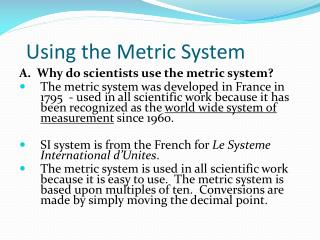DownloadDownload PresentationUsing the Metric System

# Using the Metric System

Télécharger la présentation## Using the Metric System

- - - - - - - - - - - - - - - - - - - - - - - - - - - E N D - - - - - - - - - - - - - - - - - - - - - - - - - - -
##### Presentation Transcript

1. Using the Metric System A. Why do scientists use the metric system? • The metric system was developed in France in 1795 - used in all scientific work because it has been recognized as the world wide system of measurement since 1960. • SI system is from the French for Le Systeme International d’Unites. • The metric system is used in all scientific work because it is easy to use. The metric system is based upon multiples of ten. Conversions are made by simply moving the decimal point.

2. What is the basic unit of length? • The meter – a little longer than a yard

3. What do scientists use to measure the length of an object smaller than a yard? • A centimeter – one hundredth of a meter, so there are 100 centimeters in a meter • A millimeter – There are 1,000 millimeters in a meter

4. How do scientists measure long distances? • The kilometer – There are 1,000 meters in a kilometer

5. Which measurement to USE?

6. Base Units (Fundamental Units) QUANTITY NAME SYMBOL _______________________________________________ Length meter m ----------------------------------------------------------------------------- Mass gram g ------------------------------------------------------------------------------- Time second s ------------------------------------------------------------------------------- Temperature Kelvin k -------------------------------------------------------------------------------- Volume(liquid)__________liter_____________L________________

7. SI Prefixes Prefix Symbol Multiplication Factor Term Micro u (0.000 001) one millionth Milli m (0.001) one thousandth Centi c (0.01) one hundredth Deci d (0.1) one tenth One Unit 1 one Dekadk10 ten Hecto h 100 one hundred Kilo k 1000 one thousand Mega M 1 000 000 one million

8. Metric Units Used In This Class QUANTITY NAME SYMBOL Length meter m centimeter cm millimeter mm kilometer km Mass gram g kilogram kg centigram cg milligram mg Volume liter (liquid) L (l) milliliter (liquid) mL (ml) cubic centimeter (solid) cm3

9. Derived Units • Base Units – independent of other units-measure • Derived Units – combination of base units-calculated Examples • density  g/L mass / volume (grams per liter) • volume  m x m x m = meters cubed • Velocity  m/s (meters per second

10. SCIENTIFIC NOTATION • Scientific Notation: Easy way to express very large or small numbers • A.0 x 10x • A – number with one non-zero digit before decimal • x -exponent- whole number that expresses the number decimal places • if x is (-) then it is a smaller -left • if x is (+) than it is larger-right

11. PRACTICE • Convert to Normal Convert to SN • 2.3 x 1023 m 3,400,000, 3.4 x 10-5 cm .0000000456

12. Multiplying • Calculating in Scientific notation • Multiplying- • Multiple the numbers • Add the exponents • (2.0 x 104) (4.0 x 103) = 8.0 x 107

13. Dividing • divide the numbers • subtract the denominator exponent from the numerator exponent • 9.0 x 107 3.0 x 102 • 3.0 x 105

14. Add • Add or subtract • get the exponents of all # to be the same • calculate as stated • make sure the final answer is in correct scientific notation form • 7.0 x 10 4 + 3.0 x 10 3 = • 7. 0 x 104 + .3 x 104 = 7.3 x 104 • 70,000 + 3,000 = 73000= 7.3 x104

15. subtract • 7.0 x 10 4 - 3.0 x 10 3 = • 7.0x 104 – .30 x 104 = 6.7 x 104 • 70,000 - 3 000 =67,000

16. PRACTICE • Add: • 2.3 x 103 cm + 3.4 x 105 cm • Subtract: •   2.3 x 103 cm - 3.4 x 105 cm • Multiply: • : 2.3 x 103 cm X 3.4 x 105 cm • Divide: • : 2.3 x 103 cm / 3.4 x 105 cm

17. Making Unit Conversions • Make conversions by moving the decimal point to the left or the right using: “ king henry died unitdrinking chocolate milk” Examples • 10.0 cm = __________m • 34.5 mL = __________L • 28.7 mg = __________kg

18. Factor label method /Dimensional analysis • Use equalities to problem solve converting units. • quantity desired = • quantity given x conversion factor (equality) • A-given unit • B-desired unit • C-given unit •  A x B • C B • C must equal 1 use equality sheet

19. Equalities You Need To Know 1 km = 1000 m 1 m = 100 cm 1 m = 1000 mm 1L = 1000 mL 1kg = 1000g 1 g = 100cg 1 g = 1000 mg

20. ENGLISH TO METRIC • 1 inch=2.5 centimeters • 1 gal=3.8 liters • 1lb= 4.4 Newtons • 1qt = .94 Liters • 1 ft = .30 meters • 12 in = .30 meters • 1 mi = 1.6 Km

21. Four-step approach When using the Factor-Label Method it is helpful to follow a four-step approach in solving problems: 1.What is question – How many sec in 56 min 2. What are the equalities- 1 min = 60 sec 3. Set up problem (bridges) 56 min 60 sec 1 min 4. Solve the math problem -multiple everything on top and bottom then divide 56 x 60 / 1